## I. Large margin classification:

a. Optimization objective:

There's one more algorithm that is very powerful and is very widely used both within industry and academia, and that's called the support vector machine. And compared to both logistic regression and neural networks, the Support Vector Machine, or SVM sometimes gives a cleaner, and sometimes more powerful way of learning complex non-linear functions.

We can modify logistic regression a bit, and get what is essentially the support vector machine.

So in logistic regression, we have our familiar form of the hypothesis there and the sigmoid activation function shown on the right.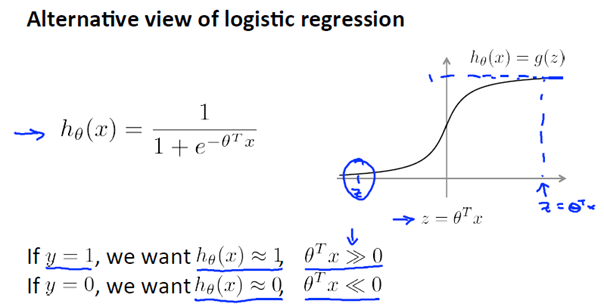If you look at the cost function of logistic regression, what you'll find is that each example (x,y) contributes a term like this to the overall cost function.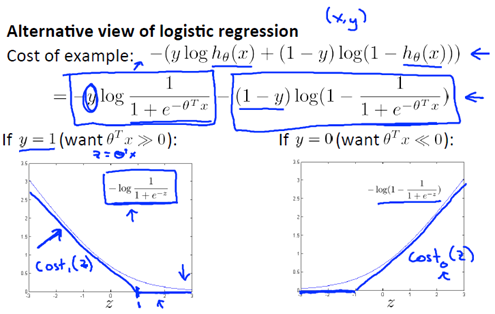That's the new cost function we're going to use for when y is equal to one, and we can imagine it should do something pretty similar to logistic regression.

But turns out, that this will give the support vector machine computational advantages and give us, later on, an easier optimization problem that would be easier for software to solve.

So what we have for the support vector machine is a minimization problem of: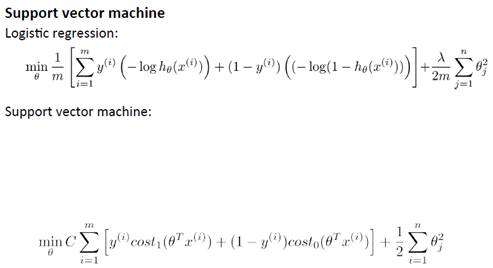So that gives us our overall optimization objective function for the support vector machine. And if you minimize that function, then what you have is the parameters learned by the SVM.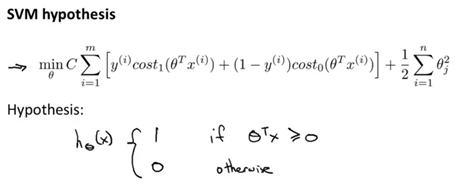Finally unlike logistic regression, the support vector machine doesn't output the probability is that what we have is we have this cost function, that we minimize to get the parameter's data, and what a support vector machine does is it just makes a prediction of y being equal to one or zero, directly.

So the hypothesis will predict one if theta transpose x is greater or equal to zero, and it will predict zero otherwise and so having learned the parameters theta, this is the form of the hypothesis for the support vector machine.

b. Large margin intuition: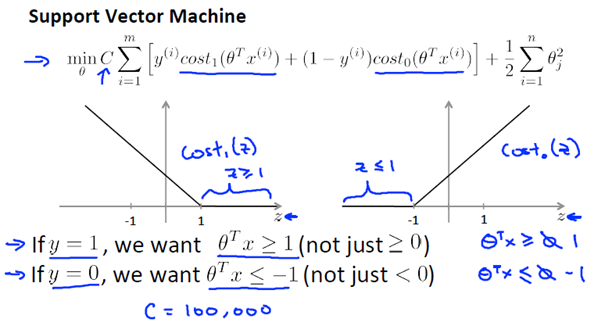Concretely, we will consider a case where we set this constant C to be a very large value, may be a hundred thousand, some huge number.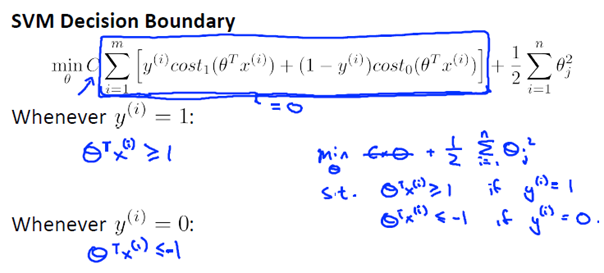If C is very,very large, then when minimizing this optimization objective, we're going to be highly motivated to choose a value, so that this first term is equal to zero.  It turns out that when we solve this optimization problem, when we minimize this as a function of the parameters theta we get a very interesting decision boundary.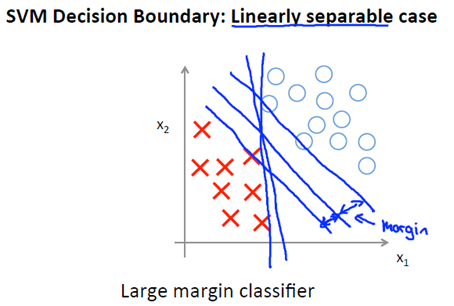The Support Vector Machines will instead choose this decision boundary, which is drawing in black. And that seems like a much better decision boundary than either of the ones that is drawn in magenta or in green. The black line seems like a more robust separator, it does a better job of separating the positive and negative examples.

And mathematically, what that does is, this black decision boundary has a larger distance.

That distance is called the margin, when we draw up this two extra blue lines, we see

that the black decision boundary has some larger minimum distance from any of the training examples, whereas the magenta and the green lines they come awfully close to the training examples.and then that seems to do a less a good job separating the positive and negative classes than the black line.

And so this distance is called the margin of the support vector machine and this gives the SVM a certain robustness, because it tries to separate the data with as a large a margin as possible. So the support vector machine is sometimes also called a large margin classifier and this is actually a consequence of the optimization problem .

SVM try to separate the positive and negative examples with as big a margin as possible.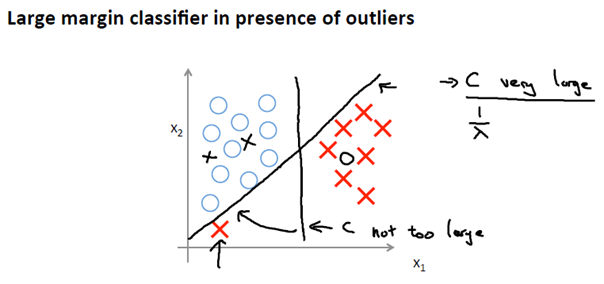So, if the regularization parameter C were very large, then SVM will change the decision boundary from the black to the magenta one but if C were reasonably small we still end up with this black decision boundary.

In practice when applying support vector machines, when C is not very very large it can do a better job ignoring the few outliers. And also do fine and do reasonable things even if the data is not linearly separable.

## II. Kernels: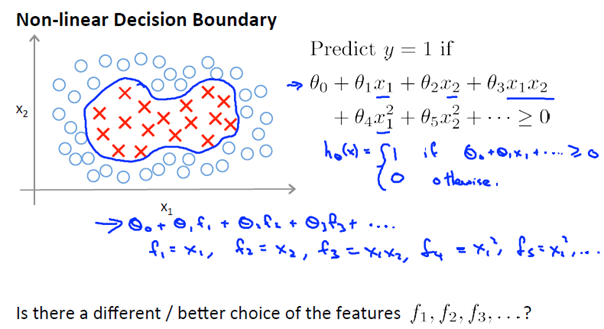So, is there a different or a better choice of the features that we can use to plug into this sort of hypothesis form. So, here is one idea for how to define new features f1, f2, f3.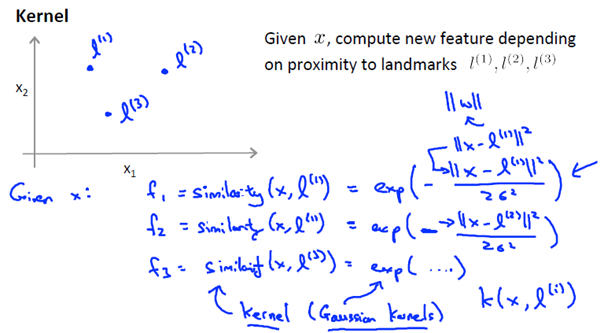On this line I am going to define only three new features, but for real problems we can get to define a much larger number. We will manually pick a few points and then call them l(1), l(2), l(3).

This particular choice of similarity function is called a Gaussian kernel. These different similarity functions are called kernels and we can have different similarity functions but the specific example here is called the Gaussian kernel.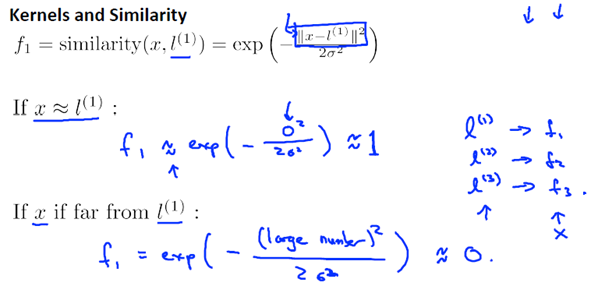So these features measure how similar X is from one of the landmarks and the feature f is going to be close to one when X is close to the landmark and is going to be 0 or close to zero when X is far from the landmark.

Each landmark defines a new feature f1, f2 and f3.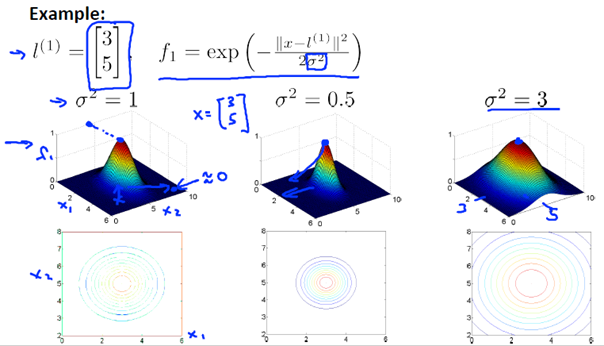In this slide we show the effects of varying this parameter sigma squared. So, sigma squared is the parameter of the Gaussian kernel and as you vary it, you get slightly different effects. We set sigma square to 0.5, what you find is that the kernel looks similar, except for the width of the bump becomes narrower. The contours shrink a bit too. The feature f1 falls to zero much more rapidly and conversely.  And if sigma squared is large, then as you move away from l1, the value of the feature falls away much more slowly.